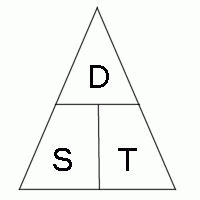# What is the speed of a car (km/hr) if it travels 16km in 13 minutes?Distance = Speed * Time
Speed = Distance / Time
Time = Distance / Speed

The Problem Apply the above formula: Speed = Distance / Time

Speed = 16km / (13min/ 60 min) --> 60min = 1hr (your statement)

Speed = 16km/1 * 60/13
(Division by a fraction is done by multiplying the dividend by the reciprocal of the divisor, in accordance with the identity)

Speed = 960 / 13 (simplify)

Speed = 73.84615385 km/hr (solution)
thanked the writer.
That's an impossible question to answer if you don't write the time elapsed during those 16 km.
thanked the writer.
Mark Mottian commented
The time elapsed was 13 minutes!!